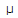# Electronics - Quantities and Units

### Exercise :: Quantities and Units - General Questions

11.

The number 14.8 can also be expressed as

 A. 1.48 × 10–1 B. 1.48 × 100 C. 1.48 × 101 D. 1.48 × 102

Answer: Option C

Explanation:

No answer description available for this question. Let us discuss.

12.

The digits in a measured number that are known to be correct are called

 A. accuracy digits B. significant digits C. error digits D. precision digits

Answer: Option B

Explanation:

No answer description available for this question. Let us discuss.

13.

Which of the following is expressed in engineering notation?

 A. 470 × 105 B. 82 × 10–2 C. 9.1 × 10–6 D. 14.7 × 108

Answer: Option C

Explanation:

No answer description available for this question. Let us discuss.

14.

The unit for frequency is the

 A. hertz B. ampere C. watt D. second

Answer: Option A

Explanation:

No answer description available for this question. Let us discuss.

15.

Add 21 mA and 8000A and express the result in milliamperes.

 A. 21.8 mA B. 218 mA C. 29 mA D. 290 mA

Answer: Option C

Explanation:

No answer description available for this question. Let us discuss.

#### Current Affairs 2021

Interview Questions and Answers